# Two Port Network: Parameters And Examples

## What is Two Port Network?

A two port network is an electrical network model with one pair of input terminals and one pair of output terminals. It is commonly used to model the voltage and current characteristics of complex electrical networks.

The figure below shows a two port network.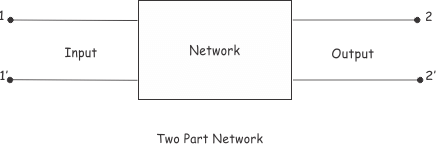A single phase transformer is an ideal example of two port network.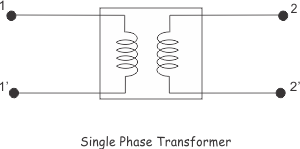When an electrical signal is applied across the input ports, there would be an electrical signal across output ports.

The relation between input and output signals of the network can be determined by transferring various network parameters, such as, impedance, admittance, voltage ratio and current ratio. Let us refer to the figure below,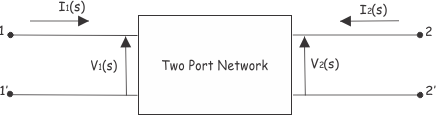Here in the network,
The transfer voltage ratio function is,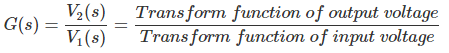The transfer current ratio function is,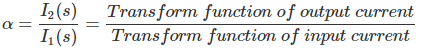The transfer impedance function is,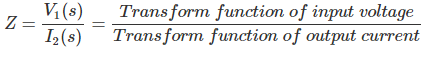The transfer admittance function is,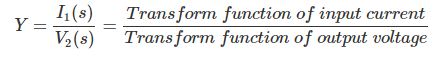## Two Port Network Parameters

There are various parameters needed to analyze a two port network. For examples, Z parameters, Y parameters, h parameters, g parameters, ABCD parameters etc.
Let us discuss these network parameters one by one to gain a better understanding of their application and uses.

## Z Parameters

Z parameters are also known as impedance parameters. When we use Z parameter for analyzing two part network, the voltages are represented as the function of currents. So,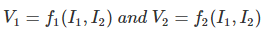The Z parameters are,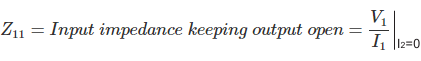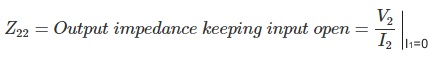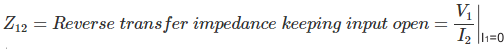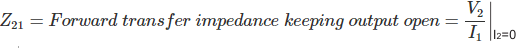The voltages are represented as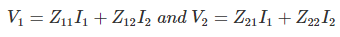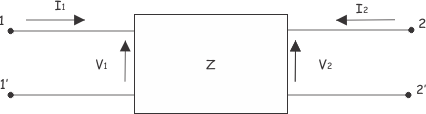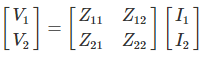## Y Parameters

Y parameter is dual of Z parameter.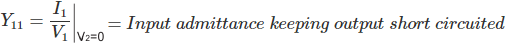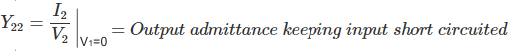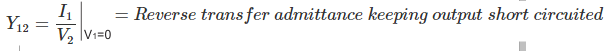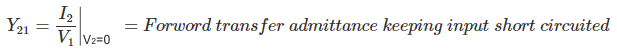In the two part network represented by admittance, the current and voltage related by the following equations,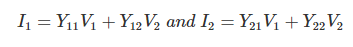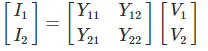## h Parameter

h parameters also known as hybrid parameters.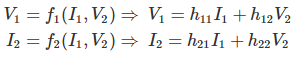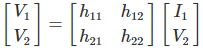In hybrid parameter circuit, voltage gain, current gain, impedance and admittance are used to determines relation between current and voltage of two port network.
Hence,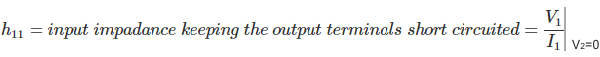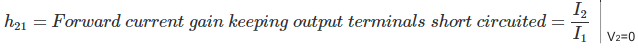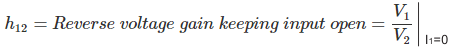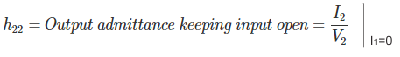## ABCD Parameter

These are also called transmission parameters. Here, voltage and current and of input part are expressed in term of output part.
Here,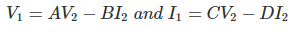In matrix form it can be written as,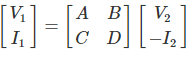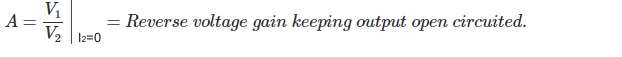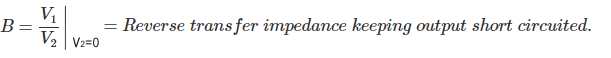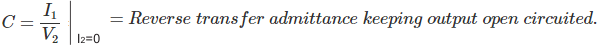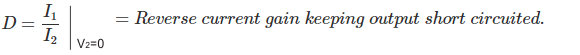Want To Learn Faster? 🎓
Get electrical articles delivered to your inbox every week.
No credit card required—it’s 100% free.